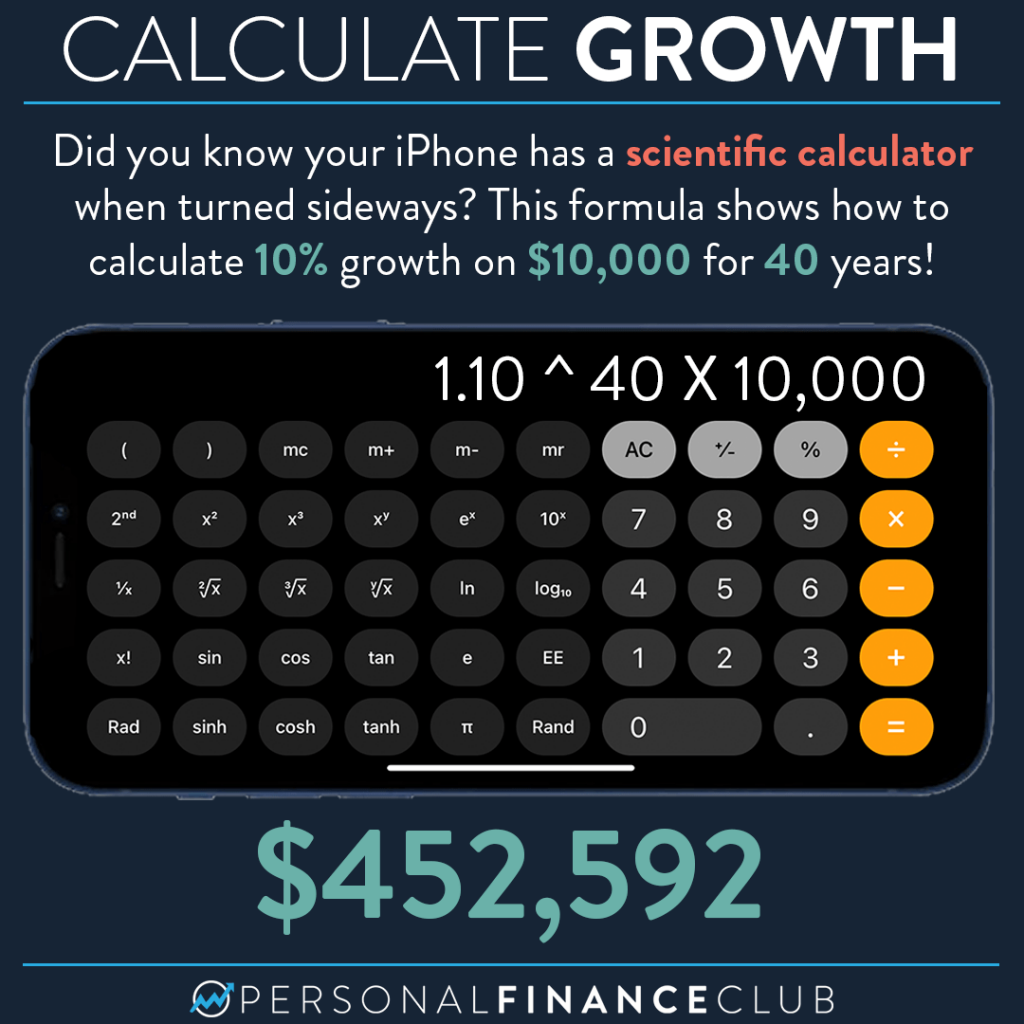# How do I calculate investment growth on my iPhone?Sometimes people ask me how to calculate the numbers I use in my examples. Well, it depends on the example. Sometimes I download historical stock or index prices into a spreadsheet and model the growth. Often it’s using an online compound growth calculator. But for something simple, you can do it right on your calculator!‎

Here’s how this formula works: If you want to grow a number by 10% you multiply it by 1.1. So 10% growth on 10,000 is 10,000 X 1.1 = 11,000.‎

If you want to grow it by 10% TWICE, you just multiply it by 1.1 again. So two years of 10% growth on 10,000 would be 10,000 X 1.1 X 1.1 = 12,100.‎

Note that you can rewrite 1.1 X 1.1 as 1.1 ^ 2. (The power operator ^ is shown on the calculator as xʸ). ‎

So 10% growth of 10,000 for two years is 10,000 X 1.1 ^ 2. So if you want to do 40 years, just enter the formula shown in this example!‎

Did you know about the scientific calculator on your iPhone?! Is this too much math!? ‎

Thanks to @mattyj48 for the idea!‎

As always, reminding you to build wealth by following the two PFC rules: 1.) Live below your means and 2.) Invest early and often.‎

-Jeremy‎

via Instagram